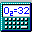##Capillary Flow and Mass Rate Calculations

### Volumetric Flow Rate Calculations

This window can be used to predict the flow rate of liquid in both open tubular and packed capillaries (aka columns). Capillaries are small tubes used in liquid chromatography, capillary electrophoresis, and other analytical chemistry fields. It is useful to be able to compute the expected linear velocity and volumetric flow rate of liquid through capillaries. The flow rate will change based on the applied pressure, the length of the capillary, the inner diameter of the capillary, and the solvent viscosity (typically 0.0089 poise). Each of these values may be changed on the page, using various units, and the correct flow rate will be computed. Choose the "View Explanatory Equations" tab to see the equations used.

When a capillary is filled with small particles, it is termed a "packed capillary". The addition of the particles results in a decreasing of the flow rate. To see the equations used under these conditions, change to Packed Capillary mode (the upper left dropdown box) and choose "View Explanatory Equations". When dealing with packing particles, you need to enter the particle diameter, and the interparticle porosity (epsilon), a "fudge factor" that accounts for the percentage of the capillary that becomes filled by the particles, thus impeding flow. A typical value for epsilon is 0.4

In addition to predicting volumetric flow rate, you can also determine what pressure, column length, or column inner diameter will be needed to give a desired volumetric flow rate. Lastly, you can enter an observed dead time (the time for solvent to travel the entire length of the column) and the program will determine what volumetric flow rate and back pressure will result in the given dead time. Use the second dropdown box on the upper left to change the computation mode.

The following table illustrates which values are used to calculate which result in the various modes. U means a User-entered value, and Calc means a calculated value. In the program (and below), text boxes highlighted in yellow are the values that the program will be computing for you, and thus, cannot be manually changed.

 Mode Back Pressure Column Length Column Inner Diameter Solvent Viscosity Particle Diameter Volumetric Flow Rate Column Dead Time Find Back Pressure Calc U U U U U Calc Find Column Length U Calc U U U U Calc Find Inner Diameter U U Calc U U U Calc Find Volumetric Flow Rate U U U U U Calc Calc Find Flow Rate using Dead Time Calc U U U U Calc U

### Mass Flow Rate Calculations

The Capillary Flow Calculator can assist in determining the Mass Flow Rate of a compound in the column and the moles of a compound injected onto a column. The Mass Flow Rate is simply the number of moles of a compound that pass by a given point on the column in one second. When injecting a "plug" of sample onto a column using a pressure-based injection, the total moles of compound injected can be computed by entering the sample concentration, flow rate, and injection time.

The concentration can be entered as a Molarity, or as a mg/mL type concentration. When using mg/mL, the program needs to know the molecular of the compound. You can either use the molecular weight of the currently selected formula in the main program window, or enter a custom numerical mass.